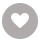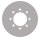# Learning Spark SQL电子书

45人正在读 | 0人评论9.8

• 读书简介
• 目录
• 累计评论(0条)
• 读书简介
• 目录
• 累计评论(0条)

Title Page

Learning Spark SQL

Credits

www.PacktPub.com

Why subscribe?

Customer Feedback

Preface

What this book covers

What you need for this book

Who this book is for

Conventions

Customer support

Errata

Piracy

Questions

Getting Started with Spark SQL

What is Spark SQL?

Introducing SparkSession

Understanding Spark SQL concepts

Understanding Resilient Distributed Datasets (RDDs)

Understanding DataFrames and Datasets

Understanding the Catalyst optimizer

Understanding Catalyst optimizations

Understanding Catalyst transformations

Introducing Project Tungsten

Using Spark SQL in streaming applications

Understanding Structured Streaming internals

Summary

Using Spark SQL for Processing Structured and Semistructured Data

Understanding data sources in Spark applications

Selecting Spark data sources

Using Spark with relational databases

Using Spark with MongoDB (NoSQL database)

Using Spark with JSON data

Using Spark with Avro files

Using Spark with Parquet files

Defining and using custom data sources in Spark

Summary

Using Spark SQL for Data Exploration

Introducing Exploratory Data Analysis (EDA)

Using Spark SQL for basic data analysis

Identifying missing data

Computing basic statistics

Identifying data outliers

Visualizing data with Apache Zeppelin

Sampling data with Spark SQL APIs

Sampling with the DataFrame/Dataset API

Sampling with the RDD API

Using Spark SQL for creating pivot tables

Summary

Using Spark SQL for Data Munging

Introducing data munging

Exploring data munging techniques

Pre-processing of the household electric consumption Dataset

Computing basic statistics and aggregations

Augmenting the Dataset

Executing other miscellaneous processing steps

Pre-processing of the weather Dataset

Analyzing missing data

Combining data using a JOIN operation

Munging textual data

Processing multiple input data files

Removing stop words

Munging time series data

Pre-processing of the time-series Dataset

Processing date fields

Defining a date-time index

Using the TimeSeriesRDD object

Handling missing time-series data

Computing basic statistics

Dealing with variable length records

Converting variable-length records to fixed-length records

Extracting data from "messy" columns

Preparing data for machine learning

Pre-processing data for machine learning

Creating and running a machine learning pipeline

Summary

Using Spark SQL in Streaming Applications

Introducing streaming data applications

Building Spark streaming applications

Implementing sliding window-based functionality

Joining a streaming Dataset with a static Dataset

Using the Dataset API in Structured Streaming

Using output sinks

Using the Foreach Sink for arbitrary computations on output

Using the Memory Sink to save output to a table

Using the File Sink to save output to a partitioned table

Monitoring streaming queries

Using Kafka with Spark Structured Streaming

Introducing Kafka concepts

Introducing ZooKeeper concepts

Introducing Kafka-Spark integration

Introducing Kafka-Spark Structured Streaming

Writing a receiver for a custom data source

Summary

Using Spark SQL in Machine Learning Applications

Introducing machine learning applications

Understanding Spark ML pipelines and their components

Understanding the steps in a pipeline application development process

Introducing feature engineering

Creating new features from raw data

Estimating the importance of a feature

Understanding dimensionality reduction

Deriving good features

Implementing a Spark ML classification model

Exploring the diabetes Dataset

Pre-processing the data

Building the Spark ML pipeline

Using StringIndexer for indexing categorical features and labels

Using VectorAssembler for assembling features into one column

Using a Spark ML classifier

Creating a Spark ML pipeline

Creating the training and test Datasets

Making predictions using the PipelineModel

Selecting the best model

Changing the ML algorithm in the pipeline

Introducing Spark ML tools and utilities

Using Principal Component Analysis to select features

Using encoders

Using Bucketizer

Using VectorSlicer

Using Chi-squared selector

Using a Normalizer

Retrieving our original labels

Implementing a Spark ML clustering model

Summary

Using Spark SQL in Graph Applications

Introducing large-scale graph applications

Exploring graphs using GraphFrames

Constructing a GraphFrame

Basic graph queries and operations

Motif analysis using GraphFrames

Processing subgraphs

Applying graph algorithms

Analyzing JSON input modeled as a graph

Processing graphs containing multiple types of relationships

Understanding GraphFrame internals

Viewing GraphFrame physical execution plan

Understanding partitioning in GraphFrames

Summary

Using Spark SQL with SparkR

Introducing SparkR

Understanding the SparkR architecture

Understanding SparkR DataFrames

Using SparkR for EDA and data munging tasks

Exploring structure and contents of Spark DataFrames

Running basic operations on Spark DataFrames

Executing SQL statements on Spark DataFrames

Merging SparkR DataFrames

Using User Defined Functions (UDFs)

Using SparkR for computing summary statistics

Using SparkR for data visualization

Visualizing data on a map

Visualizing graph nodes and edges

Using SparkR for machine learning

Summary

Developing Applications with Spark SQL

Introducing Spark SQL applications

Understanding text analysis applications

Using Spark SQL for textual analysis

Preprocessing textual data

Using word lists

Creating data preprocessing pipelines

Understanding themes in document corpuses

Using Naive Bayes classifiers

Developing a machine learning application

Summary

Using Spark SQL in Deep Learning Applications

Introducing neural networks

Understanding deep learning

Understanding representation learning

Introducing deep learning in Spark

Introducing CaffeOnSpark

Introducing DL4J

Introducing TensorFrames

Working with BigDL

Tuning hyperparameters of deep learning models

Introducing deep learning pipelines

Understanding Supervised learning

Understanding convolutional neural networks

Using neural networks for text classification

Using deep neural networks for language processing

Understanding Recurrent Neural Networks

Introducing autoencoders

Summary

Tuning Spark SQL Components for Performance

Introducing performance tuning in Spark SQL

Understanding DataFrame/Dataset APIs

Optimizing data serialization

Understanding Catalyst optimizations

Understanding the Dataset/DataFrame API

Understanding Catalyst transformations

Visualizing Spark application execution

Exploring Spark application execution metrics

Using external tools for performance tuning

Cost-based optimizer in Apache Spark 2.2

Understanding the CBO statistics collection

Statistics collection functions

Filter operator

Join operator

Build side selection

Understanding multi-way JOIN ordering optimization

Understanding performance improvements using whole-stage code generation

Summary

Spark SQL in Large-Scale Application Architectures

Understanding Spark-based application architectures

Using Apache Spark for batch processing

Using Apache Spark for stream processing

Understanding the Lambda architecture

Understanding the Kappa Architecture

Design considerations for building scalable stream processing applications

Building robust ETL pipelines using Spark SQL

Choosing appropriate data formats

Transforming data in ETL pipelines

Implementing a scalable monitoring solution

Deploying Spark machine learning pipelines

Understanding the challenges in typical ML deployment environments

Understanding types of model scoring architectures

Using cluster managers

Summary

01

David Julian
￥138.00
02

Alex Chow
￥168.00
03

James Denton
￥123.00
04

Vipul A M
￥138.00
05

Ashwin Pajankar
￥123.00
06

Altaf Hussain
￥123.00
07

Chris Halverson
￥123.00
08

David Gonzalez
￥123.00
09

Steven F. Daniel
￥138.00
10

### Learning Go Web Development￥92.00

Nathan Kozyra
￥92.00
•关注我们

最受欢迎的阅读产品

关注我们：

•关于我们

欢迎反馈宝贵意见给我们

客服书吧：当当云阅读问答